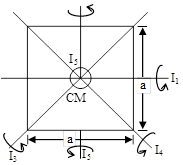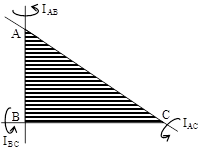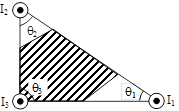# Circular Motion & Rotational Dynamics

#### Formulae For The Moment of Inertia of Regular Bodies

 SPECIAL POINT (a) Moment of inertia of square plate I1 = I3 = I4 =, I5 =(b) Momentum of inertia of cube I1 =I2 =(c) In a triangle, M.I. will be maximum relative to smallest side If AC > BC > AB, IAC > IBC > IAB(d) In triangle , M.I. will be maximum relative to that perpendicular axis which passes through least angle. If θ1 < θ2 < θ3, I1 > I2 > I3(e) Greater the mass away from axis of rotation, more will be MI

If you want to give information about online courses to other students, then share it with more and more on Facebook, Twitter, Google Plus. The more the shares will be, the more students will benefit. The share buttons are given below for your convenience.

#### IIT (Class X)

• Unit, Dimension & Error
• Vectors
• Motion in One Dimension
• PROJECTILE MOTION
• NEWTON'S LAWS OF MOTION & FRICTION
• WORK, POWER, ENERGY & CONSERVATION LAWS
• CIRCULAR MOTION & ROTATIONAL DYNAMICS
• GRAVITATION